# AGGREGATE FORMATION

This operator was defined in Klug (1982) and subsequently in Ozsoyoglu, Ozsoyoglu, & Matos (1987).

The aggregate formation operator first partitions tuples of relation R such that tuples having the same X component are in the same partition. Then the function f is applied to component A of tuples in each partition, and the X-value and the associated aggregate value are output for each partition.

Let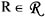be a relation included in the set of relations in the database, with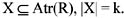Let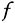be an aggregate function and A be simple-valued, with A Atr (R). Then,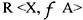is a relation with degree k+1 and is defined as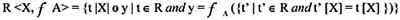where ‘o’ denotes concatenation.Multidimensional Databases: Problems and Solutions
ISBN: 1591400538
EAN: 2147483647
Year: 2003
Pages: 150